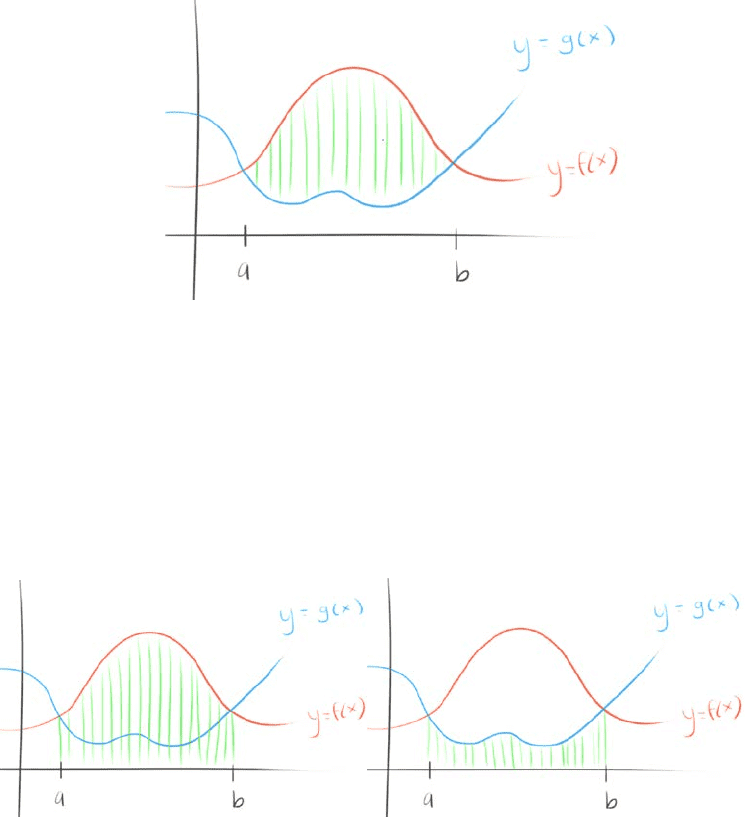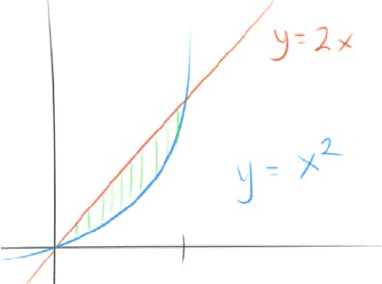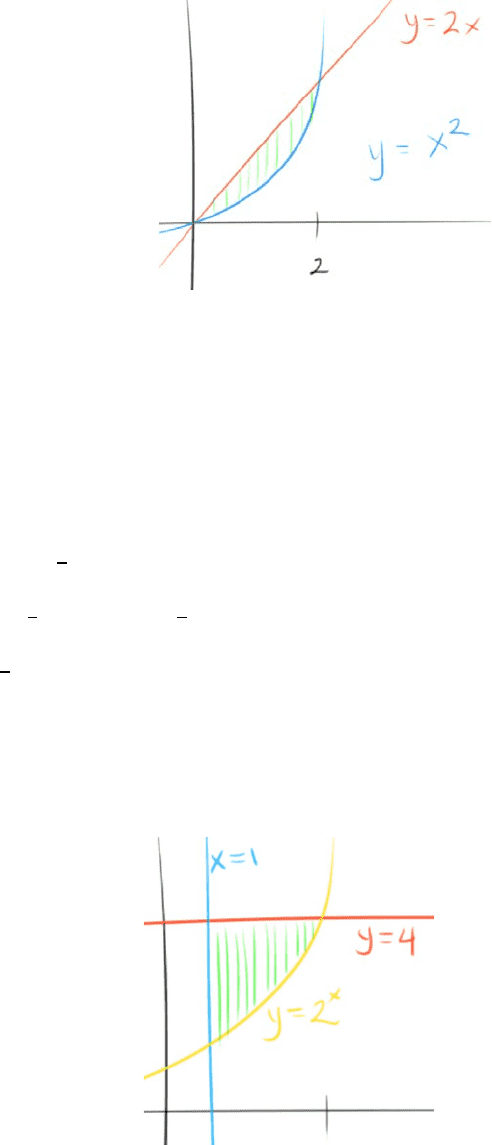Study Guides (390,000)
US (220,000)
UC-Irvine (3,000)
MATH (600)
MATH 2B (100)
All (60)
Midterm

# MATH 2B Study Guide - Fall 2018 Midterm Notes - Geta (footwear)Premium

Department
Mathematics
Course Code
MATH 2B
Professor
All
Study Guide
Midterm

This preview shows pages 1-2. to view the full 8 pages of the document.Section 6.1 Area between Curves
Find the Area of the Region
What we want to do is take the integral, which would give us the area under the curve
However, if we were to take the integral of () or () only, you wouldn’t quite get
the area highlighted in green that we want
What to do?
Take the area of () ()
Why? Because the area under the curve of () subtracted from the area under
the curve of () would give us the desired area

Only pages 1-2 are available for preview. Some parts have been intentionally blurred.

Subscribers OnlyWhat would this give us?
=
() ()
=
() ()
In other words, the area between the curves is the area of the top curve
minus the bottom curve
Example #1
Find the area between = 2 and = 2
Firstly, we want to define the boundaries that we will take the area under
Find the intersection points of the two functions
Set the functions equal to each other to do so
2 = 2
0 = 2 2
0 = ( 2)
= 2, = 0

Unlock to view full version

Subscribers Only

Only pages 1-2 are available for preview. Some parts have been intentionally blurred.

Subscribers OnlySo we will take the integral from [0, 2]
2
02 2
We know that we can compile the new integral like so because from the graph,
we can clearly see that = 2 is clearly on the top
= 2
02 2
= 2(1
33)
(221
323) (021
303)
= 4
3
Example #2
Find the area between the curves = 4, = 2, = 1
Define the boundaries
2 = 4
= 2